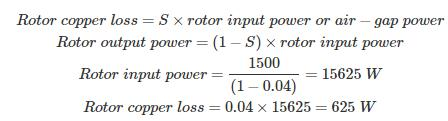Courses

# Test: Induction Machine - 2

## 25 Questions MCQ Test Electrical Engineering SSC JE (Technical) | Test: Induction Machine - 2

Description
This mock test of Test: Induction Machine - 2 for Electrical Engineering (EE) helps you for every Electrical Engineering (EE) entrance exam. This contains 25 Multiple Choice Questions for Electrical Engineering (EE) Test: Induction Machine - 2 (mcq) to study with solutions a complete question bank. The solved questions answers in this Test: Induction Machine - 2 quiz give you a good mix of easy questions and tough questions. Electrical Engineering (EE) students definitely take this Test: Induction Machine - 2 exercise for a better result in the exam. You can find other Test: Induction Machine - 2 extra questions, long questions & short questions for Electrical Engineering (EE) on EduRev as well by searching above.
QUESTION: 1

Solution:
QUESTION: 2

Solution:
QUESTION: 3

### The rotor slots are slightly skewed in squirrel - cage induction motor to

Solution:
QUESTION: 4

The frame of an induction motor is usually made of

Solution:
QUESTION: 5

The rotor of an induction motor never runs at synchronous speed, because then the relative speed between the rotating flux and rotor will be

Solution:
QUESTION: 6

A wound rotor motor is mainly used in applications where ..............

Solution:
QUESTION: 7

Which dc motor characteristic is similar to that of a 3- phase induction motor?

Solution:
QUESTION: 8

Slip ring induction motors are less extensively used than squirrel cage motors because .........

Solution:
QUESTION: 9

Which of the following statement is not valid when a squirrel-cage induction motor operates under no-load?

Solution:
QUESTION: 10

If the supply voltage of 3-phase induction motor is increased two times, then, torque is .

Solution:
QUESTION: 11

The approximate efficiency of a 3-phase, 50 Hz, 4-pole induction motor running at 1350 r.p.m. is..............

Solution:

Correct ans is A .
efficiency of motor is = Nr/Ns*100
Nr =1350
Ns= 1500
efficiency = 1350/1500*100=90

QUESTION: 12

When the supply frequency of a three phase induction motor is increased, then its synchronous speed is

Solution:
QUESTION: 13

In a double squirrel cage induction motor, the outer cage winding has ................

Solution:
QUESTION: 14

Very large 3-phase induction motors (> 25 h.p.) are started ............

Solution:
QUESTION: 15

For the same kVA rating, the leakage flux in induction motor is ....... that of transformer

Solution:
QUESTION: 16

In induction motor, greater the number of poles

Solution:
QUESTION: 17

The no-load speed of an induction motor depends upon

Solution:
QUESTION: 18

The operation of an induction motor is based on

Solution:
QUESTION: 19

The frequency of the induced emf in an induction motor is

Solution:
QUESTION: 20

At no load induction motor has possible power factor as

Solution:
QUESTION: 21

The rotor power output of 3-phase induction motor is 15 KW. The rotor copper losses at a slip of 4% will be

Solution:QUESTION: 22

The frequency of the induced emf in an induction motor is

Solution:
QUESTION: 23

A capacitor start single phase induction motor will usually have a power factor of

Solution:
QUESTION: 24

Which part will surely tell that given motor is DC motor and not an AC type?

Solution:

All other parts except brushes and commutator are same in AC machine when outer looks are only taken in consideration. Commutator is used only in DC machine for providing mechanical rectification and not in AC machine.

QUESTION: 25

Slip ring of an induction motor is usually made up of

Solution:
• A slip ring is an electromechanical device that allows the transmission of power and electrical signals from a stationary to a rotating structure.
• It is used in case of AC supply, where  AC is required.
• With slip ring, the voltage in the external circuit varies like a sine wave and the current alternates the direction.
• Phosphor bronze is an alloy of copper, tin, and phosphorus. The tin increases the corrosion resistance and strength of the alloy. The phosphorus increases the wear resistance and stiffness of the alloy.
• Phosphorous bronze provide good electrical conductivity and low thermal conductivity
• Since slip ring induction motors used to accelerate heavy loads over a  long period of time and to withstand excessive heat phosphorous bronze slip is used.